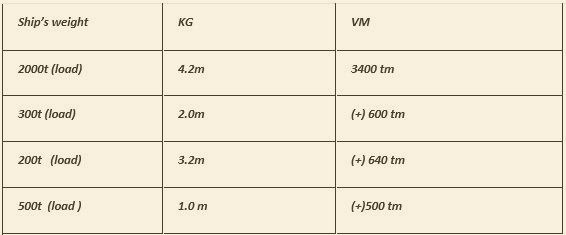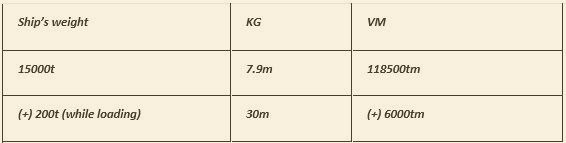top of pageSearch

# Chapter 7

### A ship of displacement 2000t and KG 4.2m, loads 300t 0f cargo (KG 2.0m ), 200t of cargo (KG 3.2m) and 500t of bunkers ( KG 0m ). Find her final KG.Final W = 300t Final VM = 10140 tm

Final KG = (Final VM) / (Final W) Final KG = 10140 / 3000 = 3.38m

### 2. A ship of displacement 3000t and KG 3.9m, loads cargo and follows :- 200t in N0.1 LH ( KG 3.0m )300t on the deck ( KG 6.4m ),150t and NO.3 TD (KG 5.2m ) and 350t in NO.4 LH (KG 4.0m ). Find the final KG .

Solution:Final W = 4000 t Final VM = 16400 tm

Final KG = (Final VM) / (Final W) Final KG = (16400/4000) = 4.1m

### 3. A ship of load displacement 10,000 t, KG 6.0m, discharge cargo of 250t ( KG 3.0m ) and 150t ( KG 4.0m). Find her final KG.

Solution :Final W = 9600t Final VM = 58650 tm

Final KG = (Final VM) / (Final W) Final KG = (58650 / 9600) = 6.109m.

### 5. Ship of 2000t displacement of KG 3.66m , loads 1500t( KG5.5m ) 3500t (KG 4.60m ), and takes 1520t of bunkers (KG 0.60m ). She discharge 2000t cargo ( KG 2.44m ). and consume 900t of bunkers ( KG 0.40m ). Find the KG at the and of the voyage.

Solution :Final W = 5620t Final VM = 27342tm

Final KG = (Final VM) / (Final W) Final KG = (27342) / (5620) = 4.865m

### 6. A ship of 3200t displacement KG 6.2m , loads 5200t of cargo (KG 4.8 ). Find the amount of deck cargo (KG 10.2m ) that can be loaded so that the KG shall be 6.0m when loading is completed .

Solution:Final W = (8400 + X )t Final VM =(44800 +10X);

Final KG = (Final VM) / (Final W) 6 = (44800 + 10X )/( 8400 + X) 6 (8400 + X ) = ( 44800 +10X) (50400 + 6X ) = (10X + 44800) ( 10X – 6X) = (50400 – 44800) 4X = 5600 X = 1400t

Hence , amount of deck cargo to be loaded to get required KG is 1400 t

### 7. A ship 0f 2600t displacement , KG 4.88m, loads of 4600 t of homogeneous cargo (KG 5.0m ). Find how much deck cargo (KG 10m ) may be loaded to obtain a final KG of 5.11m.

Solution:Final W = (7200 + X) t Final VM =(35688 + 10X) tm

Final KG = (Final VM) / (Final W) 5.11 = (35688 + 10X )/( 7200 + X ) 5.11( 7200 + X) = (35688 + 10X) (36792 + 5.11X ) = (35688 +10X) (36792 – 35688) = (10X – 5.11X ) X = 1104 / 4.89 X =225.766t.

Hence amount of deck cargo added to get desired KG is 225.76 t.

### 8. A heavy-lift derrick is used to discharge a 100t package from a ship of displacement 8000t kg 8.2m . If the KG of the weight while on board is 3m and if the derrick head is 25m above the keel , find the kg of the ship (a) while discharging and( b ) after discharging

Solution :

CASE 1Final W = 8000t Final VM = 67800t

Final KG = (Final VM) / (Final W) Final KG = ( 67800/8000) = 8.475m

Case – 2Final W = 7900t Final VM = 65300tm

Final KG = (Final VM) / (Final W) Final KG = (65300/7900) = 8.266m

### 9. On a ship of 15000t displacement kg 7.9m , a weight of 200t is loaded on the UD (KG 12m ) above the Find the KG of the ship (a) while loading , and (b) After loading .

Solution :

Case – 1Final W = 15200T Final VM = 124500tm

Final KG = (Final VM) / (Final W) Final 1.KG = (124500/15200) = 8.190m

Case – 2Final W = 15200 Final VM = 120900 tm

Final KG = (Final VM) / (Final W) Final KG = (120900/15200) =7.954m.

### 10. On a ship of 11000 t displacement KG7.2m a shore crane is used to shift a heavy-lift from the UD (KG 12m to the LH (KG 3m ) . Find the kg of the ship (a) during shifting is (b) after shifting .

Solution :

Case -1Final W = 10820t Final VM = 77040 tm

Final KG = (Final VM) / (Final W) Final KG = 77040 / 10820 = 7.120m

Case – 2Final W = 11000 Final VM = 77580tm

Final KG = (Final VM) / (Final W) Final KG = (77580/11000) = 7.053m.